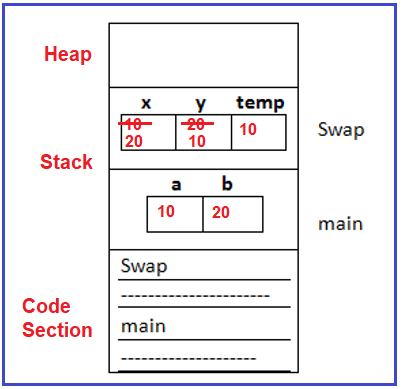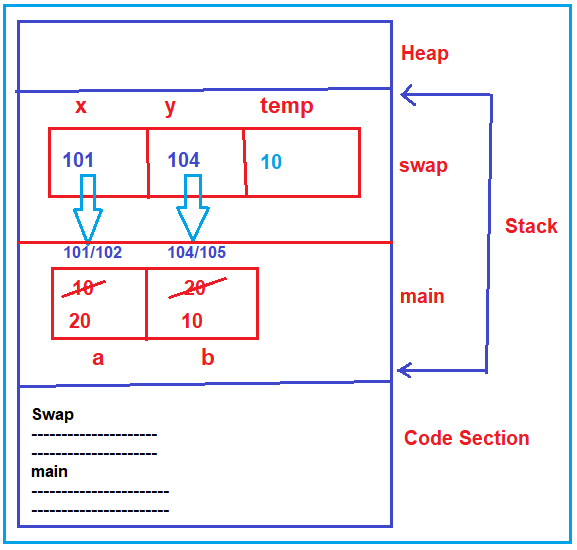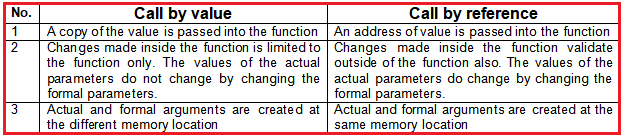# Call by Value and Call by Address in C

## Function Call by Value and Call by Address in C Language

In this article, I am going to discuss Functions Call by Value and Call by Address in C Language with Examples. Please read our previous articles, where we discussed the Types of User-Defined Functions in C Language with Examples. At the end of this article, you will understand the following pointers in detail.

1. Parameter Passing Methods in C
2. Pass by value (or) call by value
3. How does Call by Value work?
4. When do we need to use Pass by Value?
6. How does call by address work?
7. How to call a Function in C Program?
8. What is Function Call by Value in C?
9. What is Function Call by Address in C?
10. Difference between call by value and call by Address in c
11. Understanding Return by Value and Return by Address
##### Parameter Passing Methods in C Language

In this article, we will learn the Parameter Passing Methods i.e. the 2 parameter passing methods (pass by value and pass by address). In order to explain these two parameter passing methods, we have taken 1 simple example which is the swapping of numbers. So, let us understand these two methods one by one.

##### Pass by value (or) call by value:

In order to understand pass by value or call by value please have a look at the below example. As you can see in the below example, the main function has variables ‘a’ and ‘b’ with the values 10 and 20 respectively. Then the main function calls the swap function. The swap function takes 2 parameters x and y. Then the swap function swaps the numbers x and y with the help of a temporary variable. Then the control comes back to the main function and it will print the values of ‘a’ and ‘b’.

```#include <stdio.h>
void swap (int x, int y)
{
int temp;
temp = x;
x = y;
y = temp;
}

int main ()
{
int a, b;
a = 10;
b = 20;
swap (a, b);
printf ("%d %d", a, b);
}
```
##### How does Call by Value Work in C Language?

In the main function, while calling the swap function we pass two parameters i.e. a and b, and these parameters are called actual parameters. The swap function which takes x and y parameters is called formal parameters.

When the main function is called the swap function, the value of x (i.e. 10) and y (i.e. 20) are copied to the x and y variables respectively. Here, the formal parameters are normal variables so take the values. As the swap function does not return anything so the return type is void. Within the swap function, we have implemented the logic to swap the values of x and y variables using the temp variable.

temp = x; this will store 10 in the temp variable
x = y; this line will store 20 in the x variable
y = temp; here it will store 10 in the y variable.

So, here the formal parameters (x and y) are modified but actual parameters (a and b) remain the same. The swapping is done inside the variables of the swap function only which are not reflected inside the actual parameters of the main function.

When the swap function completes its execution, it comes back to the main function from where it is being called. Then inside the main function, it prints the value of a and b and you can see 10 and 20 on the console window. For a better understanding please have a look at the below image.So, in the pass-by-value mechanism, any changes are done to formal parameters that will not reflect in the actual parameter.

##### When do we need to use Pass by Value?

When we don’t want to modify the actual parameters, then we can use pass by value method. Even though you can also use it when the method returns some value.

So, the swap function should not be done using a pass-by-value. If you want to add two numbers and return the result then in such cases you can use pass by value. But here it is also not suitable for swapping two numbers.

##### Pass by Address (or) Call By Address Mechanism in C Language

In the call by address mechanism, the addresses of the actual parameters are passed to formal parameters and the formal parameters must be pointers. Any changes that are done with the formal parameters will modify the same with the actual parameters. Now, we need to understand two things, how to write call by value or call by address and how it works?

Note: The point that you need to remember is, call by address uses pointers.

##### Example to Understand Pass by Address (or) Call By Address in C Language

In order to understand the call by address, please have a look at the below example and observe the syntaxes carefully. This is the same swapping example using the call by address. As you can see in the below code, inside the main function while calling the swap function, we are passing the addresses of the actual parameters (i.e. &a and &b), not the values (i.e. 10 and 20). This is the first change i.e. passing the addresses. We know that pointer variables can only hold addresses. So, the second change that we did is we create the formal parameters as pointer variables i.e. (*x and *y).

```#include <stdio.h>
void swap (int *x, int *y)
{
int temp;
temp = *x;
*x = *y;
*y = temp;
}

int main ()
{
int a, b;
a = 10;
b = 20;
swap (&a, &b);
printf ("%d %d", a, b);
}
```

Note: In call by address, the formal parameters must be pointers and here we have to use de-referencing that is *, for accessing the data of actual parameters, and here address will be passed in the case of actual parameters.

Now I hope the syntax is clear and let’s proceed and understand the workflow of the call by address.

##### How does call by address work in C Language?

The main function is having 2 variables ‘a’ and ‘b’ and they are having values of 10 and 20 respectively. Next, the swap function is called by the main function and the addresses are passed. In the swap function, the pointers *x and *y will take the addresses. So, now the swap function can access the variables of the main function using pointers.

Note: The point that you need to remember is one function cannot access the variables directly but it can access the variables indirectly by using pointers.

Within the swap function, we have the logic to swap the values of the temp variable. Let us see how they work?

temp = *x; this will store 10 in the temp variable. *x will point to the actual value of the address it holds i.e. a variable.
*x = *y; this line will store 20 in the “a” variable. This is because *y points to the b variable and its value is 20 and *x points to the “a” variable.
*y = temp; here it will store 10 in the b variable. This is because *y points to the b variable.

So, you can observe that the actual variables are modified. So, when the function swap function ends, the control comes back and when it prints the value of ‘a’ is 20, and the value of ‘b’ is 10 and these are swapped. For better understanding, please have a look at the following diagram.So, a call-by address is a suitable mechanism for modifying the actual parameters. We will be using this type of code more frequently in our programs. You should be familiar with this one. The call-by address is more useful. I hope you understand the basics of Call by Value and Call by Address. Let us proceed further and understand these two techniques in detail with more examples.

##### How to call a Function in C Program?

There are two ways that a C function can be called from a program. They are,

1. Call by value / Pass by Value
2. Call by reference / Pass by reference
##### What is Function Call by Value in C?

It is a concept of calling a function by sending value type data or passing the value type data to a function. In the call by value, actual arguments and format arguments both are value-type data. If any modifications are occurring to the formal argument those changes will not be affected by actual arguments.

The call by value method of passing arguments to a function copies the actual value of an argument into the formal parameter of the function. In this case, changes made to the parameter inside the function have no effect on the argument. By default, C programming uses call by value to pass arguments. In the call-by-value method, the value of the variable is passed to the function as a parameter. The value of the actual parameter cannot be modified by a formal parameter. Different Memory is allocated for both actual and formal parameters. Because the value of the actual parameter is copied to the formal parameter.

Note:

1. Actual parameter – This is the argument that is used in the function call.
2. Formal parameter – This is the argument that is used in the function definition
##### What is Function Call by Address?

It is a concept of calling the function by sending address type data or passing address type data to a function. In the call-by address, actual arguments are address type and formal arguments are pointer type. In the call by address if any modification occurs on formal arguments those changes will be affected by actual arguments.

The call by Address method of passing arguments to a function copies the address of an argument into the formal parameter. Inside the function, the address is used to access the actual argument used in the call. It means the changes made to the parameter affect the passed argument. In the call-by-reference method, the address of the variable is passed to the function as a parameter. The value of the actual parameter can be modified by a formal parameter. The same memory is used for both actual and formal parameters since the only address is used by both parameters.

Note: C programming language doesn’t support the call by reference. Call by reference is an OOPL concept that is used to access the data by using the reference type. C programming language doesn’t support reference type that’s why call by reference is not possible.

##### Difference Between Call By Value and Call By Address in C Language:##### Return by Value in C Language:

When the function is returning value type data then it is called return by value. When the function is not returning any values then specify the return type as void. Void means nothing i.e. function doesn’t return any value. When the function is returning i.e. what type of data it is returning, the same type of return statement is required to specify. In the implementation, when the function is returning an integer value, specify the return type as int, i.e. function returning value type called return by value.

##### Return by Address in C Language:

When the function is returning address type data then it is called return by address. When the function is not returning any values then specify the return type as void. When the function is returning the int value then specify the return type as int i.e. function returning value called return by value. When the function is returning an integer value address then specify the return type as an int* i.e. function returning address called return by address. The basic advantage of return by address is one function related to local data can be accessed from outside of the function.